Jet Set Go! All about Aeroplanes Jet Set Go! All about Aeroplanes

# Polygon

A polygon is a two-dimensional geometric figure that has a finite number of sides. The sides of a polygon are made of straight line segments connected to each other end to end. Thus, the line segments of a polygon are called sides or edges. The point where two line segments meet is called vertex or corners, henceforth an angle is formed. An example of a polygon is a triangle with three sides. A circle is also a plane figure but it is not considered a polygon, because it is a curved shape and does not have sides or angles. Therefore, we can say, all the polygons are 2d shapes but not all the two-dimensional figures are polygons.

We can observe different types of polygons in our daily existence and we might be using them knowingly or unknowingly. In this article, you will the meaning and definition of a polygon, types of a polygon, real-life examples of polygon shapes along with their properties and related formulas in detail.

## What are Polygons?

A Polygon is a closed figure made up of line segments (not curves) in a two-dimensional plane. Polygon is the combination of two words, i.e. poly (means many) and gon (means sides).

A minimum of three line segments is required to connect end to end, to make a closed figure. Thus a polygon with a minimum of three sides is known as Triangle and it is also called 3-gon. An n-sided polygon is called n-gon.

## Polygon shape

By definition, we know that the polygon is made up of line segments. Below are the shapes of some polygons that are enclosed by the different number of line segments.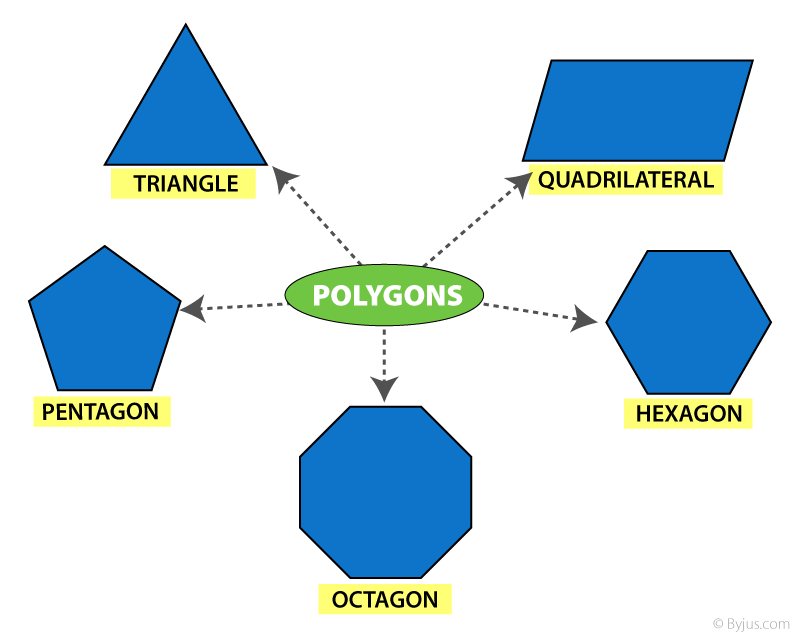## Types of Polygon

Depending on the sides and angles, the polygons are classified into different types, namely:

• Regular Polygon
• Irregular Polygon
• Convex Polygon
• Concave polygon

### Regular Polygon

If all the sides and interior angles of the polygon are equal, then it is known as a regular polygonThe examples of regular polygons are square, equilateral triangle, etc.

### Irregular Polygon

If all the sides and the interior angles of the polygon are of different measure, then it is known as an irregular polygon. For example, a scalene triangle, a rectangle, a kite, etc.

### Convex Polygon

If all the interior angles of a polygon are strictly less than 180 degrees, then it is known as a convex polygon. The vertex will point outwards from the centre of the shape.

### Concave Polygon

If one or more interior angles of a polygon are more than 180 degrees, then it is known as a concave polygon. A concave polygon can have at least four sides. The vertex points towards the inside of the polygon.

However, a number of polygons are defined based on the number of sides, angles and properties.

## Angles of Polygon

As we know, any polygon has as many vertices as it has sides. Each corner has a certain measure of angles. These angles are categorized into two types namely interior angles and exterior angles of a polygon.

### Interior Angle Property

The sum of all the interior angles of a simple n-gon = (n − 2) × 180°

Or

Sum = (n − 2)π radians

Where ‘n’ is equal to the number of sides of a polygon.

For example, a quadrilateral has four sides, therefore, the sum of all the interior angles is given by:

Sum of interior angles of 4-sided polygon = (4 – 2) × 180°

= 2 × 180°

= 360°

### Exterior angle property

The sum of interior and the corresponding exterior angles at each vertex of any polygon are supplementary to each other. For a polygon;

 Interior angle + Exterior angle = 180 degrees Exterior angle = 180 degrees – Interior angle

## Properties

The properties of polygons are based on their sides and angles.

• The sum of all the interior angles of an n-sided polygon is (n – 2) × 180°.
• The number of diagonals in a polygon with n sides = n(n – 3)/2
• The number of triangles formed by joining the diagonals from one corner of a polygon = n – 2
• The measure of each interior angle of n-sided regular polygon = [(n – 2) × 180°]/n
• The measure of each exterior angle of an n-sided regular polygon = 360°/n

## Area and Perimeter Formulas

The area and perimeter of different polygons are based on the sides.

Area: Area is defined as the region covered by a polygon in a two-dimensional plane.

Perimeter: Perimeter of a polygon is the total distance covered by the sides of a polygon.

The formulas of area and perimeter for different polygons are given below:

 Name of polygon Area Perimeter Triangle ½ x (base) x (height) a+b+c Square side2 4 (side) Rectangle Length x Breadth 2(length+breadth) Parallelogram Base x Height 2(Sum of pair of adjacent sides) Trapezoid Area = 1/2 (sum of parallel side)height Sum of all sides Rhombus ½ (Product of diagonals) 4 x side Pentagon $$\begin{array}{l}\frac{1}{4} \sqrt{5(5+2 \sqrt{5})} side^{2}\end{array}$$ Sum of all five sides Hexagon 3√3/2 (side)2 Sum of all six sides

Let us see one of the frequently used and the primary types of polygon, i.e. triangle.

## Triangles (3-gon)

A triangle is the simplest form of the polygon that has three sides and three vertices. The triangles are also classified into different types, based on the sides and angles.

 An interesting fact about the Triangle: The Sum of all the angles of the triangle is always equal to 180° (straight angle).

### Triangles – Based on Sides

• Equilateral triangle – Having all sides equal and angles of equal measure. It is also called an equiangular triangle.
• Isosceles triangle – Having any 2 sides equal and angles opposite to the equal sides are equal.
• Scalene triangle – Has all the 3 sides unequal.

See the below figure, to see the difference between the three types of triangles.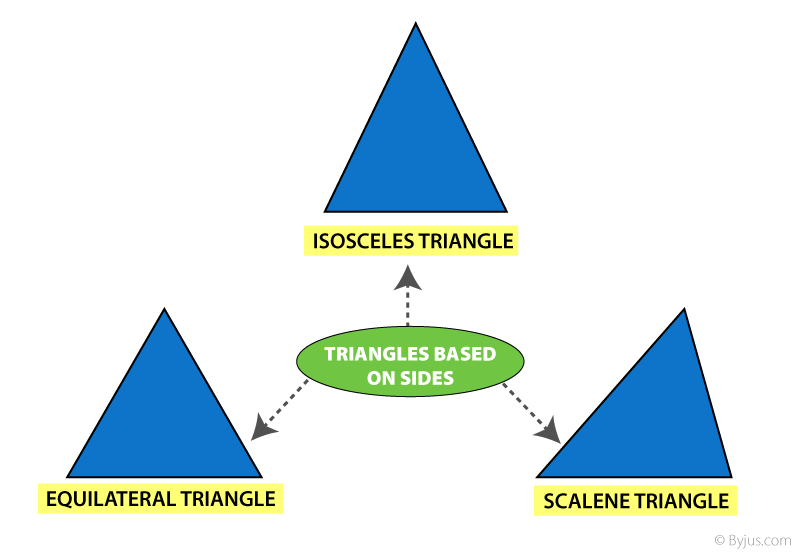### Triangles- Based on Angles

• Acute angled Triangle – Each angle is less than 90°
• Right Angled Triangle – Any one of the three angles equal to 90°
• Obtuse Angled Triangle – Any one angle is greater than 90°

The below figure shows the three types of angles, based on angles.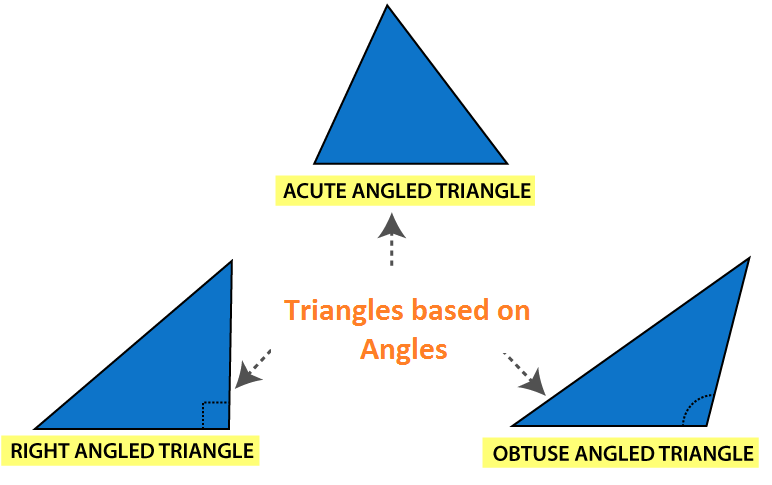### An Activity:

• Draw different triangles of any dimensions using squares and measuring scales.
• Measure the length of the sides and note them down.
• You will observe that the sum of any 2 sides of the triangle is Always Greater than the 3rd side.

A Quadrilateral is a polygon having a number of sides equal to four. That means a polygon is formed by enclosing four line segments such that they meet at each other at corners/vertices to make 4 angles.

Like triangles, a quadrilateral is also classified with different types:

The below figure shows the classification of quadrilaterals.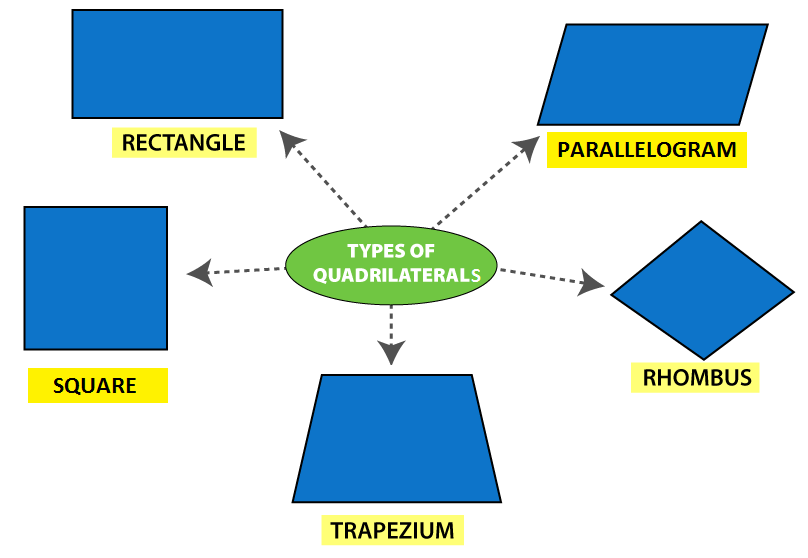The table below gives the comparison of Opposite sides, angles, and diagonals of different Quadrilaterals.

 Quadrilateral Opposite Sides All sides Equal Opposite angles equal Diagonal Diagonal Parallel Equal Equal Perpendicular Rectangle ✔ ✔ ✖ ✔ ✔ ✔ Parallelogram ✔ ✔ ✖ ✔ ✖ ✖ Rhombus ✔ ✔ ✔ ✔ ✖ ✔ Trapezium ✖ (Only one side) ✖ ✖ ✖ ✖ ✖ Square ✔ ✔ ✔ ✔ ✔ ✔

## Names of Polygon

 Polygon No. of Sides No. of Diagonal No. of vertices Interior Angle Triangle 3 0 3 60 Quadrilateral 4 2 4 90 Pentagon 5 5 5 108 Hexagon 6 9 6 120 Heptagon 7 14 7 128.571 Octagon 8 20 8 135 Nonagon 9 27 9 140 Decagon 10 35 10 144 Hendecagon 11 44 11 147.273 Dodecagon 12 54 12 150 Triskaidecagon 13 65 13 158.308 Tetrakaidecagon 14 77 14 154.286 Pentadecagon 15 90 15 156

## Three Dimensional Shape (3-D shape)

A three-dimensional shape is a solid object that is formed by a combination of polygons and 2d shapes. Some of the 3d shapes which we can observe in real-life are: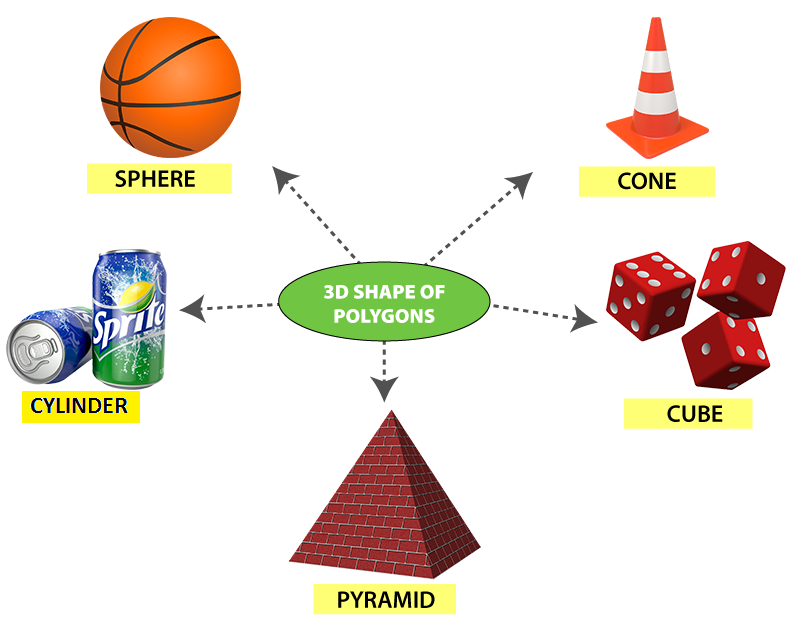• These are the shapes that can be projected on a piece of paper but cannot be drawn on paper. These shapes are known as solids.
 2-D shape (Polygon) 3-D Shape Edge Edge Vertices Vertices — Faces
• 3-D shapes have faces as the distinguishing feature.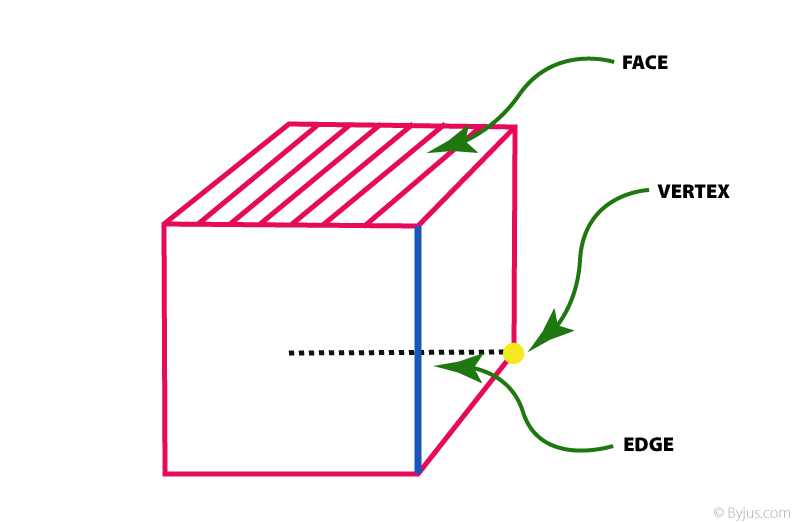To know more about polygons, types of polygons and 3-D shapes, download BYJU’S – The Learning App.

## Frequently Asked Questions – FAQs

### What is a polygon?

A polygon is a closed two-dimensional shape that is formed by enclosing line segments. A minimum of three line segments are required to make a polygon.

### What is called a polygon with 7 sides?

A polygon with 7 sides is called a Heptagon. This polygon has 7 vertices.

### What is called a polygon with 9 sides?

A polygon with 9 sides is known as Nonagon. This polygon has 9 vertices.

### How many diagonals does a polygon have?

If there are n sides in a polygon (or n-sided polygon), then the polygon will have n(n – 3)/2 diagonals. Triangles do not have diagonals.

### What are the different types of polygons?

Polygons are classified mainly into four categories. They are:
Regular polygon – all the sides and measure of interior angles are equal
Irregular polygon – all the sides and measure of interior angles are not equal, i.e. different
Convex polygon – all the interior angles of a polygon are strictly less than 180 degrees. The vertex will point outwards from the centre of the shape
Concave polygon – one or more interior angles of a polygon are more than 180 degrees. A concave polygon can have at least four sides. The vertex points towards the inside of the polygon.

1. Yuvraj Tamta

This ia a great way of learning!!!!

2. Thank you for helping me

3. hacker

I like studing with buyjus its a very nice application

4. Ashmita

I am so happy for the conceptual understanding. It has helped me to help my students

5. This is helping to me for my studies

6. Its easy understand for teachers and parents. Great workbooks.

7. Gaming YT

it is easy to understand and helps for math projects
easy to learn

8. Nandani Maurya

Byjus app very good , it is easy to understantand and helps formath